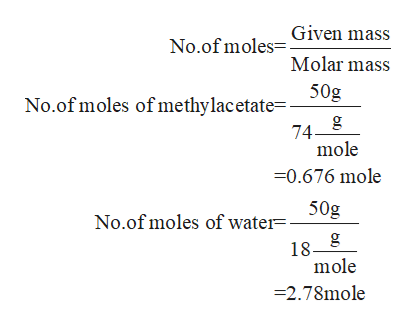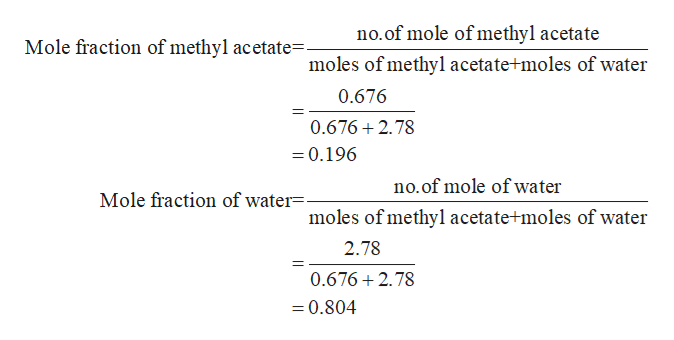# A solution of methyl acetate (CHO,) and water is boiling at 95.7°C. A sample of the vapor above the solution is cooled until it condenses. This condensedsample is analyzed, and turns out to be 50.% methyl acetate by mass.Calculate the percent by mass of methyl acetate in the boiling solution. Here's some data you may need:vapor pressureat 95.7 °Cnormaldensityboiling point2419. torr0.93mL57. °Cmethyl acetate651. torr1.00mL100. CwaterBe sure your answer has 2 significant digits.Note for advanced students: you may assume the solution and vapor above it are ideal.x10wwww.mw.?Xww.aimCheckPrivacyTerms of UseExplanation2019 McGraw-Hill Education. All Rights Reserved.

Question
104 views

Calculate the percent by mass of methyl acetate in appointing solution around two significant digits

check_circle

Step 1

Given that,

Boiling point of the solution = 95.7 C

Mass of methyl acetate = 50%

Assume that mass of the solution = 100g

Then, mass of methyl acetate = 50 g

Mass of water = 50g

Step 2

Now you calculate the no.of mole of methyl acetate and waterhelp_outlineImage TranscriptioncloseGiven mass No.of moles Molar mass 50g No.of moles of methylac etate= 74- mole 0.676 mole 50g No.of moles of water=- 18 g mole 2.78mole fullscreen
Step 3

Now, you calculate the mole fraction...help_outlineImage Transcriptioncloseno.of mole of methyl acetate Mole fraction of methyl acetate=- moles of methyl acetate moles of water 0.676 0.676 2.78 =0.196 no.of mole of water Mole fraction of water=- moles of methyl acetate+moles of water 2.78 0.676 2.78 =0.804 fullscreen

### Want to see the full answer?

See Solution

#### Want to see this answer and more?

Solutions are written by subject experts who are available 24/7. Questions are typically answered within 1 hour.*

See Solution
*Response times may vary by subject and question.
Tagged in

### Quantitative analysis